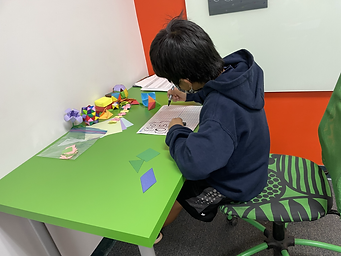## Mr. Jake

### Target 1​

###### Lesson Type:

New

Geometry

:

Coordinates

Prove geometric polygon theorems on the coordinate plane.

###### 1:

Prove that given coordinate pairs construct specific polygons using understandings of shape attributes (number of sides, angle measurements, etc.).

8th

###### Vocabulary:

Activities:

Students visualized how 2D figures could be drawn on the coordinate plane.

Students drew cards and figured out how to draw them on the coordinate plane. They recorded the coordinate points and traded with a partner to guess what figure made those points.

Students played a guessing game with coordinates that the teacher wrote on the board and discussed the patterns found in each coordinate. Students plotted the points to prove that the shape could be made with those points.### Home Exploration

###### Guiding Questions:## Absent Students:

### Target 2

:

###### 1:

Recognize that “rounding” is the identification of the multiple of ten that is the closest to the given number (10, 100, 1000, 1/10, 1/100, 1/,000, etc.).

###### 2:

Visually represent the rounding of decimals on a number line.

###### 3:

Round numbers with fractional parts, using the same value concepts used to round whole numbers.

6th

###### Vocabulary:

Round Up, Round Down, Decimal, Place Value

Activities:

Students rounded eight- to ten-digit numbers to any given place. They were given Post-It notes with different numbers and what place to round to, and they threw bean bags to determine which ones to solve. The person with the most Post-Its won the game.### Home Exploration

###### Guiding Questions:### Target 3

:

###### Vocabulary:

Activities:### Home Exploration